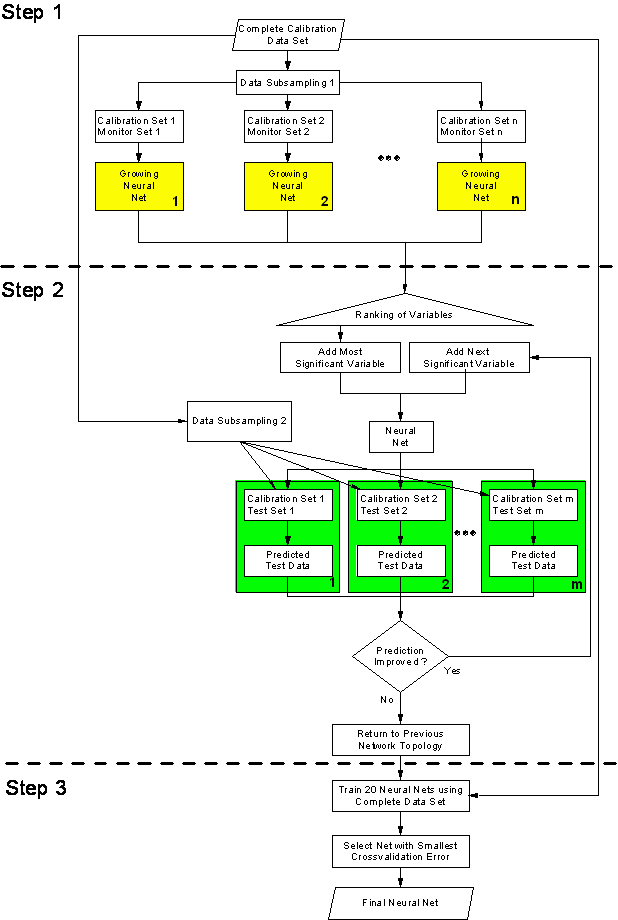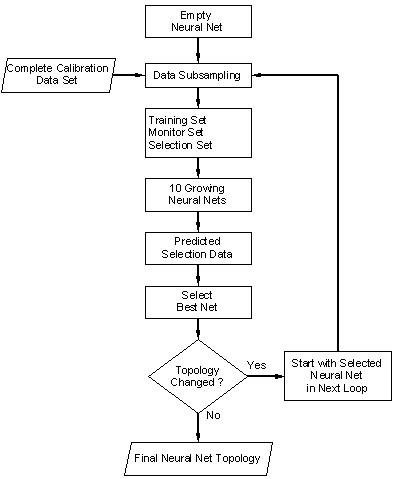Home News About Me Ph. D. Thesis Abstract Table of Contents 1. Introduction 2. Theory  Fundamentals of the Multivariate Data Analysis 3. Theory  Quantification of the Refrigerants R22 and R134a: Part I 4. Experiments, Setups and Data Sets 5. Results  Kinetic Measurements 6. Results  Multivariate Calibrations 7. Results  Genetic Algorithm Framework 8. Results  Growing Neural Network Framework 8.1. Modifications of the Growing Neural Network Algorithm 8.2. Application of the Growing Neural Networks 8.3. Growing Neural Network Algorithm Frameworks 8.4. Applications of the Growing Neural Network Frameworks 8.5. Conclusions and Comparison of the Different Methods 9. Results  All Data Sets 10. Results  Various Aspects of the Frameworks and Measurements 11. Summary and Outlook 12. References 13. Acknowledgements Publications Research Tutorials Downloads and Links Contact Search Site Map Print this Page## 8.3.   Growing Neural Network Algorithm Frameworks

The first framework for the growing neural networks is similar to the genetic algorithm framework, which was proposed in section 7.2 and will be further referred to as parallel growing neural network framework. The framework, which is presented in figure 53, can be divided into three steps. The first step consists of multiple parallel runs of the growing neural network algorithm with different training and monitor data subsets. When all networks have finished growing, the variables are ranked according to the frequency of being used in the different networks. In the second step, the algorithm builds the final neural network in an iterative procedure by adding the variables according to their rank step by step to a fully connected neural network. During each step, the performance of the neural network is evaluated by the use of different training and test data subsets. The iterative algorithm stops when the addition of the next variable does not improve the predictions of the test data subsets any more. As the number of hidden variables (organized in 1 hidden layer) is determined by the mean number of hidden variables of the networks built during the first step, the third step of the growing neural net framework, which only trains the final network with the complete calibration data set, is less complicated than the third step of the genetic algorithm framework.

The second framework is based on loops using the knowledge of previous runs of the growing algorithm and will be further referred to as loop-based framework. The concept is illustrated in figure 54. Several parallel runs of the growing neural network algorithm are performed using different random initial weights but the same data subsets for training, monitoring and selection. The network showing the best prediction of the data subset for selection is used as initial network for the growing algorithm of the next loop cycle. Thereby the algorithm starts not with an empty neural network but with the selected built network and new subsets of the data for training, monitoring and selection. This procedure is repeated, until the selected network has the same topology than the network selected in the previous loop cycle.

In both frameworks, the complete calibration data set has to be split several times into subsets for training, monitoring, testing and selection. Similar to the genetic algorithm framework, this was performed by a random subsampling procedure.figure 53:  Flow chart of the parallel growing neural network framework.figure 54:  Flow chart of the loop-based growing neural network framework.

According to the introduction to the network optimization in section 2.8.2, both frameworks follow different strategies. The parallel framework only uses the variable selection property of the growing neural networks ignoring the information of the inner topology of the grown networks. This framework is suitable for the implementation on massive parallel computer systems as there are only few nodes for information exchange. A high number of parallel runs of the algorithm allows a variable selection practically independent from the partitioning of the data set. The second framework uses the possibility of the algorithm to build non-uniform neural networks with an optimized internal topology adapted for a specific problem. In contrast to the first framework, this framework is less suitable for parallel computer systems and less partitions of the data set are used, since the algorithm typically stops after several loop cycles.

 Page 109 © Frank Dieterle, 03.03.2019